If you’ve ever used a spreadsheet, you’ll be very comfortable with how Panorama handles automatic calculations. You simply enter a formula in any field that you want to be filled with a calculation. To illustrate this we’ll use this simple database with four numeric fields for data entry (A, B, C and D) and two fields that we will be calculated (Total and Avg).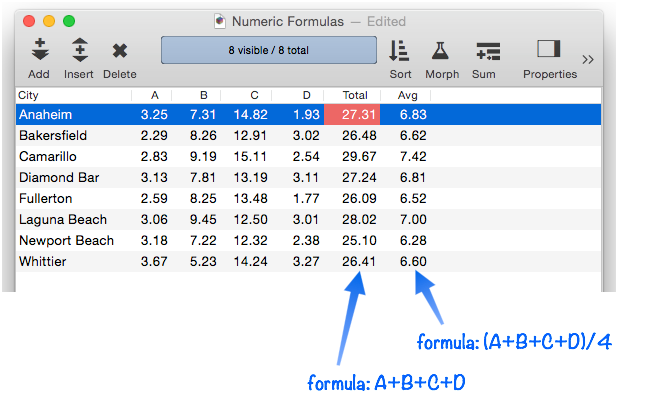Use the Field Properties Panel to set up the formula associated with a field.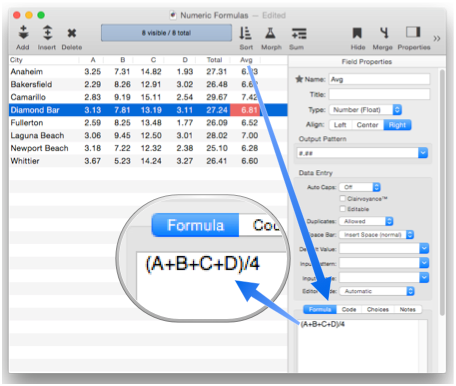With the formulas set up, the Total and Avg fields will be automatically calculated when data is entered into A, B, C or D.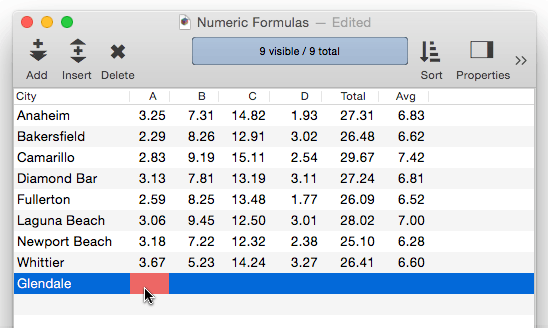When the calculations are performed, the result of the calculation is stored into the database, just as if you had typed it in. Once the calculation result is stored in the database, it is just like any other data – you can perform selections based on these results, sort based on the results, etc. For example, you could use the Sort Down command (see Sorting Data) to rank the data from the highest to lowest average.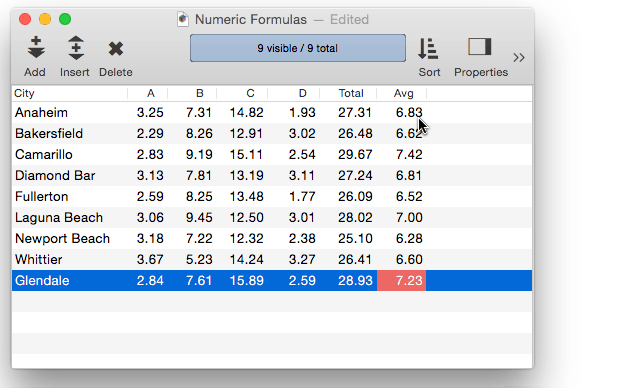Since the calculation result is actually stored in the database, you can edit it just like any other data.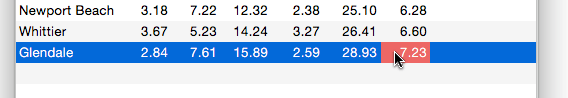Of course you probably don’t want to edit calculated results. If you want to prevent a calculated field from being edited in the data sheet, uncheck the Editable option in the Field Properties Panel.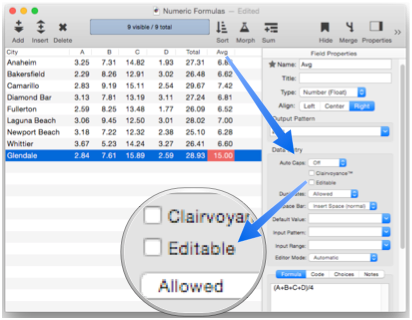#### Recalculating an Entire Column

Panorama runs the automatic calculations any time data is entered manually, but it doesn’t update the calculation when the formula is changed, or if data is changed some other way (for example by importing or using the Morph dialog. In those situations, use the Field>Morph>Recalculate Field command to re-run the formula for every selected record in the database.

To illustrate this, suppose that the D column is deleted from the example database shown above. Once the D field is deleted you’ll need to adjust the formulas to remove this field. However, simply changing the formula doesn’t update the existing data in this field – the changed formula will only come into play when new data is entered. So after deleting the field and revising the formulas, you’ll need to recalculate, as shown in this movie.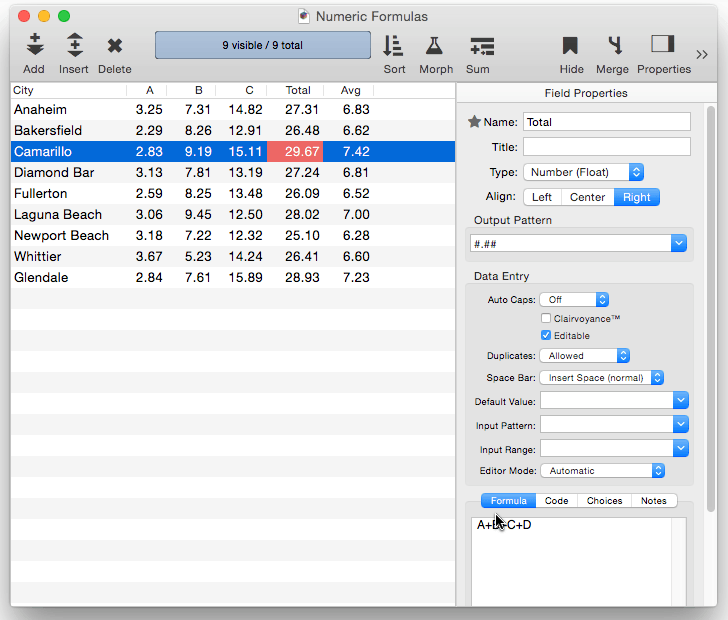#### Standalone Formulas

Unlike some other database programs, you don’t have to set up a field just to run a calculation. Panorama allows formulas to be used “bare”, not associated with any field. For example a formula can be used in various graphics objects, including Text List Object, Image Display Object, and Web Browser Object. When used this way, Panorama calculates the formula result, immediately displays the result, and then discards it. You only need to set up a formula associated with a field when you want to be able to do further operations with the calculation results, for example sorting, selecting, or analyzing.